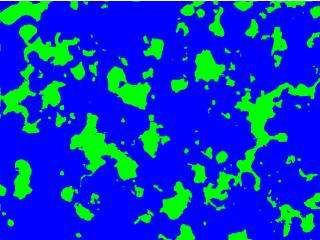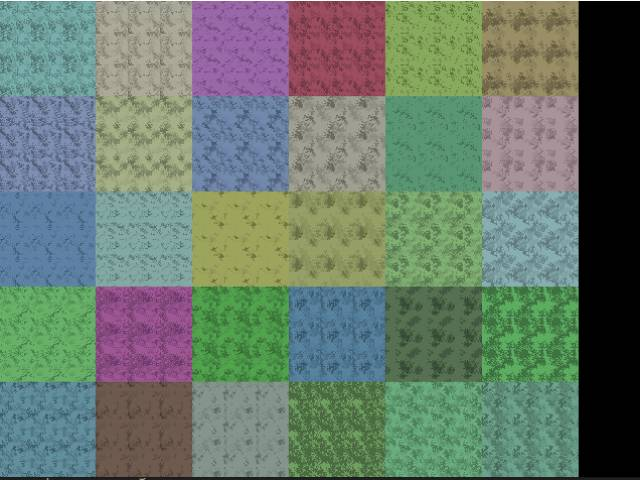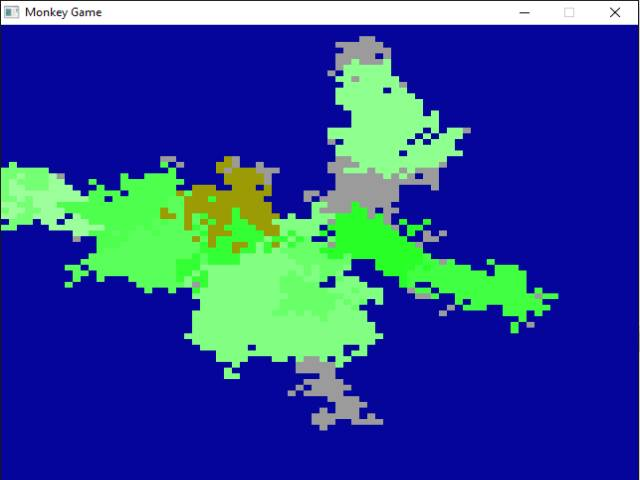-=+=- -=+=- -=+=- -=+=- -=+=- -=+=- -=+=- -=+=- -=+=- -=+=- -=+=- -=+=- -=+=- -=+=- -=+=- -=+=- -=+=- -=+=- -=+=- -=+=- -=+=- -=+=- -=+=- -=+=- -=+=- -=+=- -=+=- -=+=- -=+=- -=+=- (c) WidthPadding Industries 1987 0|668|0 -=+=- -=+=- -=+=- -=+=- -=+=- -=+=- -=+=- -=+=- -=+=- -=+=- -=+=- -=+=- -=+=- -=+=- -=+=- -=+=- -=+=- -=+=- -=+=- -=+=- -=+=- -=+=- -=+=- -=+=- -=+=- -=+=- -=+=- -=+=- -=+=- -=+=-

Pakz# Fractal Leaf (Barsley Furn)

16th February 2023(Image.jpeg)
Francois Lionet Poster this code on his facebook. Very little code for a real good looking factal leaf.
I converted it to HTML/Javascript.
[code]
&lt;!DOCTYPE html&gt;
&lt;html&gt;
&lt;meta charset=''UTF-8''&gt;
*snip*

Pakz# Raycast engine(short)(javascript/html)

23rd July 2022

Raycast engine in javascript/html

Pakz# Berzerk level generator

11th January 2022

Level generator from the classic game berzerk

Pakz# Recursive map generator

10th January 2022(Image.jpeg)
[code]
-- Recursiveprocgen
-- Use this function to perform your initial setup
function setup()
print(''Hello World!'')
mw = 40
mh = 40
tw = WIDTH/mw
th = HEIGHT/mh

createmap()
end
-- This fun*snip*

Pakz# L Systems - plant

28th October 2021

L systems - string with turtle instructions

Pakz# sprite sheet animator(bplus)

15th July 2021

A ancient tool to animate spritesheet

Pakz# Random Walker Mazelike maps(Godot script)

16th February 2021

Some Godot script for creating maps.

Pakz# Scanline fill

30th January 2021

Fast scanline fill routine in c language

Pakz# Orientation of point vs line

24th November 2019

Is a point left or center or right of a line

Pakz# Convex Hull or Gift Wrapping algorithm

21st November 2019

create lines around set of points.

Pakz# Polygon vs Polygon Collision

13th November 2019

Is one polygon colliding with another polygon

Pakz# Point versus Polygon Collision

2nd November 2019

Check if a point is inside a polygon

Pakz# Shortest turn angle vs target angle

27th October 2019

Get distance between angle and target angle. (turrets/homing missiles)

Pakz# Random Bag

16th July 2019

Get more predictable numbers

Pakz# Rivers on a top down map.

30th May 2019

Create 2d Rivers

Pakz6th April 2019Create a greyscale stone like texture

Pakz# Bitwise technique

23rd January 2019

Id System for neighbouring tiles

Pakz# Maps or oilish paintings

21st January 2019Smearing a additive brush

Pakz# Pixel Art Pine Tree(s)

21st January 2019Generate sheets of pine trees(xmas?)

Pakz# Seamless Grow Tiles(grass/desert etc)

2nd July 2018Create Tiles with zones method

Pakz# Maps - Horizontal Line Puller

20th June 2018Pull sections from a line to create maps

Pakz# Map Generator Lines and Zones(grow)

20th June 20182d Maps

Pakz# Chunk System example (2d)

23rd April 2018

Basic Chunks system example.

Pakz# 2d Metaballs

4th February 2018

Old Demoscene effect

Pakz# Horizontal Hall with Rooms Generator

28th January 2018Hall with Rooms generator

More - Older Posts